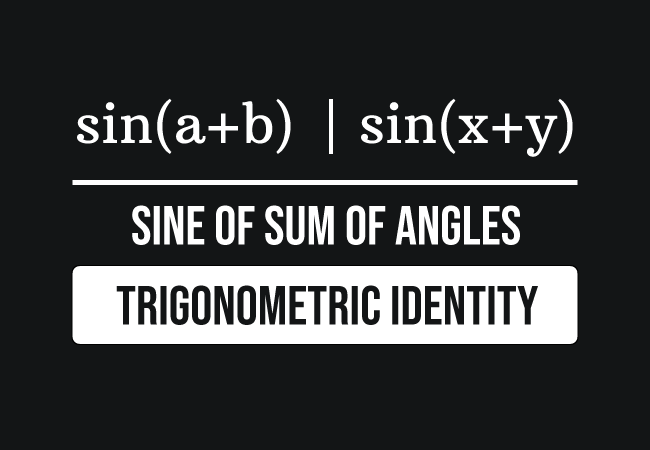# Sine angle sum identity

## Formula

$(1).\,\,$ $\sin{(a+b)}$ $\,=\,$ $\sin{a}\cos{b}$ $+$ $\cos{a}\sin{b}$

$(2).\,\,$ $\sin{(x+y)}$ $\,=\,$ $\sin{x}\cos{y}$ $+$ $\cos{x}\sin{y}$

### Introduction

Let’s assume that $a$ and $b$ are two variables, which represent two different angles. The sum of angles is written as $a+b$, which is a compound angle. The sine of a compound angle $a$ plus $b$ is written as $\sin(a+b)$ in mathematics.The sine of sum of angles $a$ and $b$ is equal to the sum of the product of sine of angle $a$ and cosine of angle $b$, and the product of cosine of angle $a$ and sine of angle $b$.

$\sin{(a+b)}$ $\,=\,$ $\sin{a} \times \cos{b}$ $+$ $\cos{a} \times \sin{b}$

This mathematical equation is called the sine angle sum trigonometric identity in mathematics.

#### Usage

The sine angle sum trigonometric identity is used in two different cases in mathematics.

##### Expansion

The sine of sum of two angles is expanded as the sum of products of sine and cosine of both angles.

$\implies$ $\sin{(a+b)}$ $\,=\,$ $\sin{(a)}\cos{(b)}$ $+$ $\cos{(a)}\sin{(b)}$

##### Simplification

The sum of products of sine and cosine of both angles is simplified as the sine of sum of two angles.

$\implies$ $\sin{(a)}\cos{(b)}$ $+$ $\cos{(a)}\sin{(b)}$ $\,=\,$ $\sin{(a+b)}$

#### Forms

The angle sum trigonometric identity in sine function is written in several forms but it is popularly expressed in the following three forms.

$(1).\,\,$ $\sin{(A+B)}$ $\,=\,$ $\sin{A}\cos{B}$ $+$ $\cos{A}\sin{B}$

$(2).\,\,$ $\sin{(x+y)}$ $\,=\,$ $\sin{x}\cos{y}$ $+$ $\cos{x}\sin{y}$

$(3).\,\,$ $\sin{(\alpha+\beta)}$ $\,=\,$ $\sin{\alpha}\cos{\beta}$ $+$ $\cos{\alpha}\sin{\beta}$

#### Proof

Learn how to derive the sine of angle sum trigonometric identity by a geometric approach in trigonometry.

Latest Math Topics
Jun 26, 2023
Jun 23, 2023

Latest Math Problems
Jul 01, 2023
Jun 25, 2023
###### Math Questions

The math problems with solutions to learn how to solve a problem.

Learn solutions

Practice now

###### Math Videos

The math videos tutorials with visual graphics to learn every concept.

Watch now

###### Subscribe us

Get the latest math updates from the Math Doubts by subscribing us.